Ex 9.5

Chapter 9 Class 12 Differential Equations
Serial order wise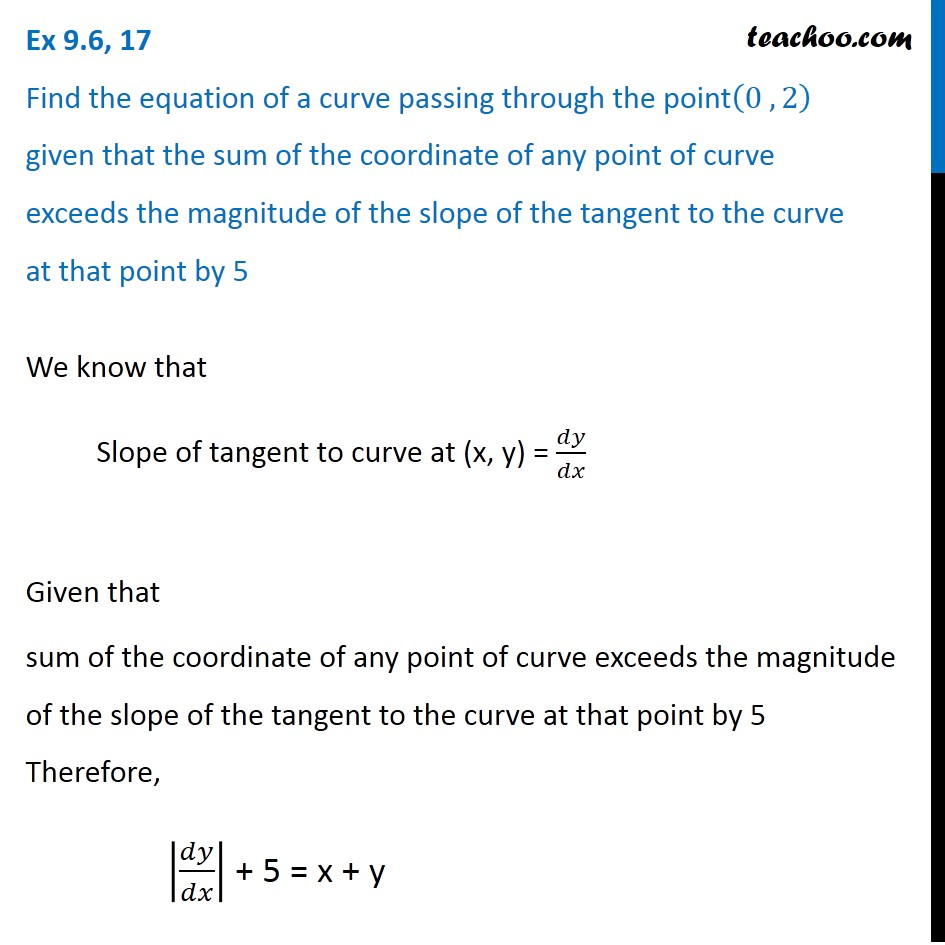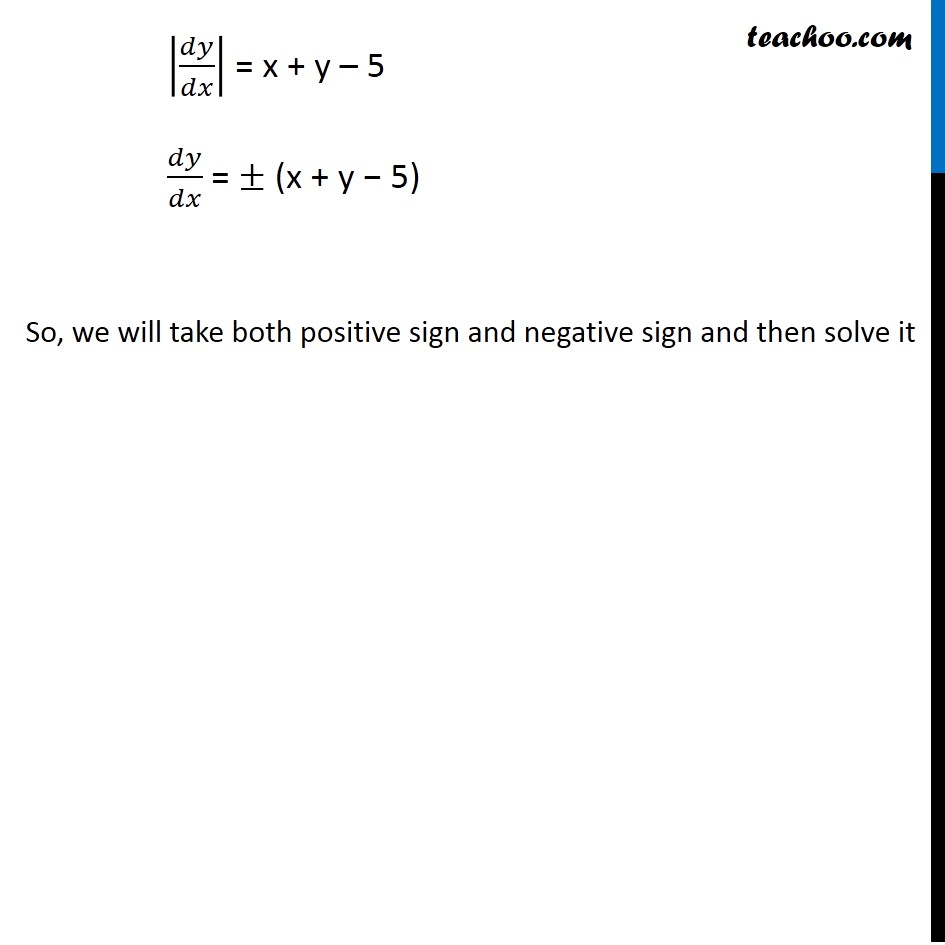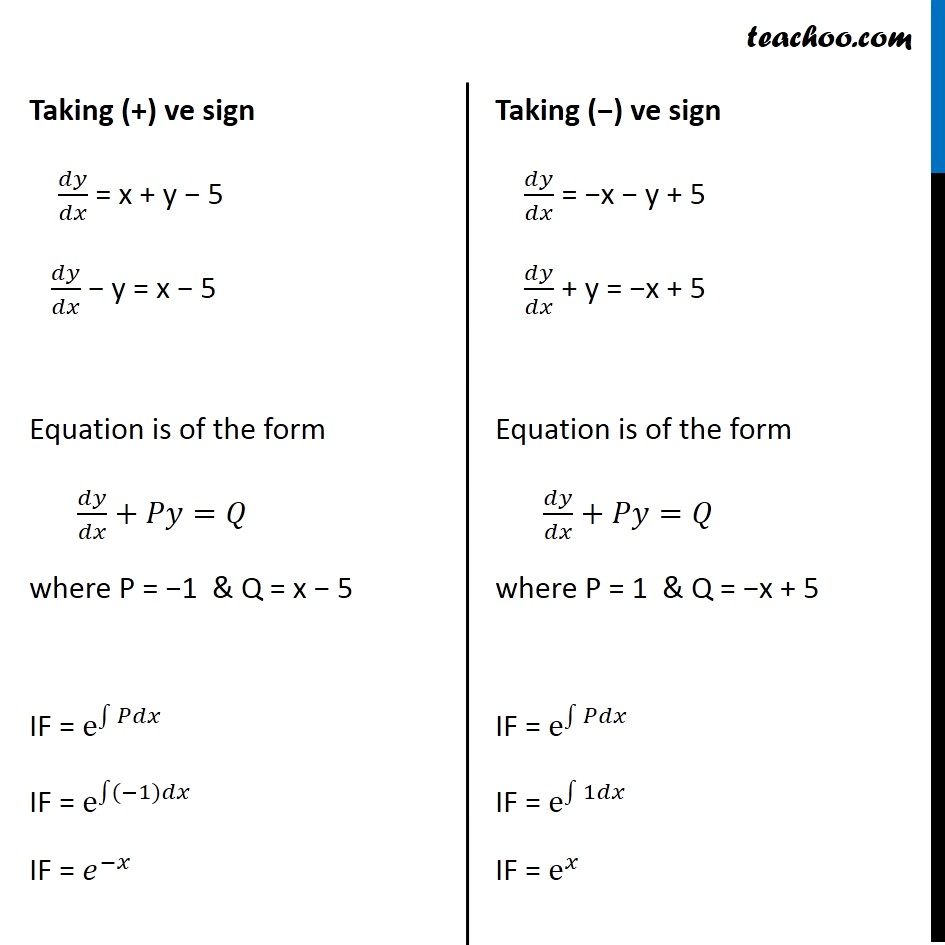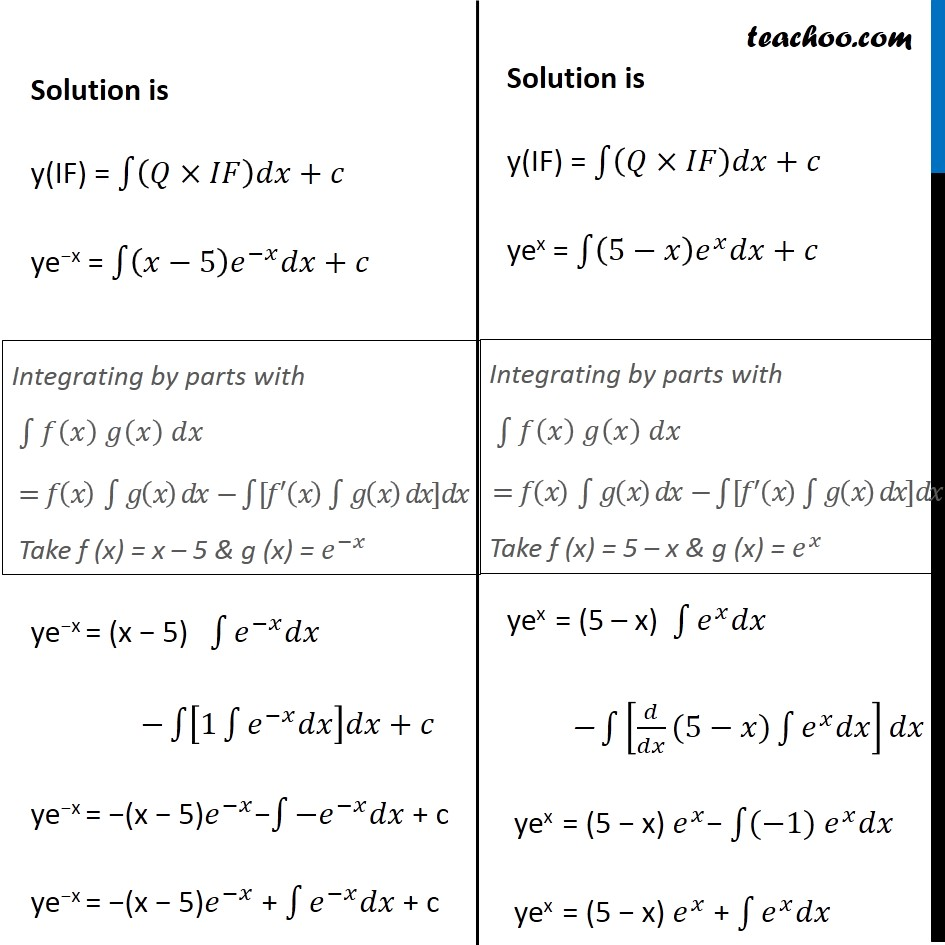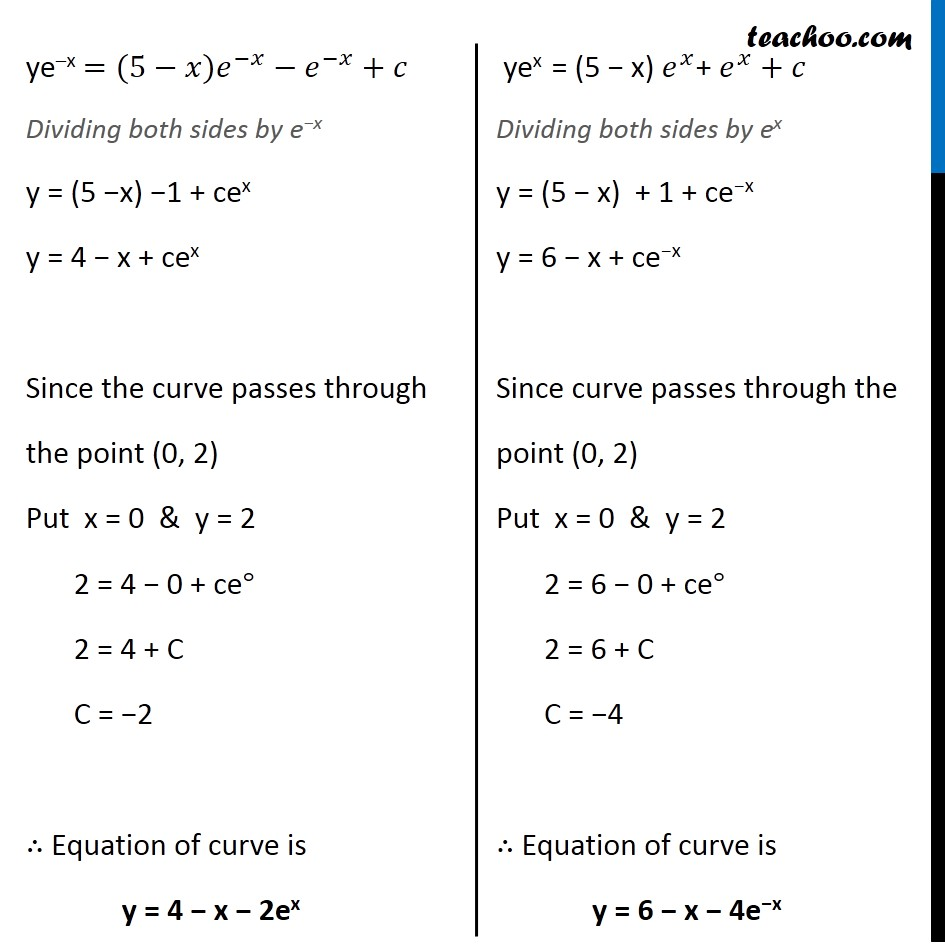Learn in your speed, with individual attention - Teachoo Maths 1-on-1 Class

### Transcript

Ex 9.5, 17 Find the equation of a curve passing through the point(0 , 2) given that the sum of the coordinate of any point of curve exceeds the magnitude of the slope of the tangent to the curve at that point by 5 We know that Slope of tangent to curve at (x, y) = 𝑑𝑦/𝑑𝑥 Given that sum of the coordinate of any point of curve exceeds the magnitude of the slope of the tangent to the curve at that point by 5 Therefore, |𝑑𝑦/𝑑𝑥| + 5 = x + y |𝑑𝑦/𝑑𝑥| = x + y – 5 𝑑𝑦/𝑑𝑥 = ± (x + y − 5) So, we will take both positive sign and negative sign and then solve it Taking (+) ve sign 𝑑𝑦/𝑑𝑥 = x + y − 5 𝑑𝑦/𝑑𝑥 − y = x − 5 Equation is of the form 𝑑𝑦/𝑑𝑥+𝑃𝑦=𝑄 where P = −1 & Q = x − 5 IF = e^∫1▒𝑃𝑑𝑥 IF = e^(∫1▒〖(−1)〗 𝑑𝑥) IF = 𝑒^(−𝑥) Taking (−) ve sign 𝑑𝑦/𝑑𝑥 = −x − y + 5 𝑑𝑦/𝑑𝑥 + y = −x + 5 Equation is of the form 𝑑𝑦/𝑑𝑥+𝑃𝑦=𝑄 where P = 1 & Q = −x + 5 IF = e^∫1▒𝑃𝑑𝑥 IF = e^∫1▒1𝑑𝑥 IF = e^𝑥 Solution is y(IF) = ∫1▒〖(𝑄×𝐼𝐹)𝑑𝑥+𝑐〗 ye−x = ∫1▒〖(𝑥−5) 𝑒^(−𝑥) 𝑑𝑥+𝑐〗 ye−x = (x − 5) ∫1▒〖𝑒^(−𝑥) 𝑑𝑥〗 −∫1▒〖[1∫1▒〖𝑒^(−𝑥) 𝑑𝑥〗]𝑑𝑥+𝑐〗 ye−x = −(x − 5)𝑒^(−𝑥)−∫1▒〖〖−𝑒〗^(−𝑥) 𝑑𝑥〗 + c ye−x = −(x − 5)𝑒^(−𝑥) + ∫1▒〖𝑒^(−𝑥) 𝑑𝑥〗 + c Solution is y(IF) = ∫1▒〖(𝑄×𝐼𝐹)𝑑𝑥+𝑐〗 yex = ∫1▒〖(5−𝑥) 𝑒^𝑥 𝑑𝑥+𝑐〗 yex = (5 – x) ∫1▒〖𝑒^𝑥 𝑑𝑥〗 − ∫1▒[𝑑/𝑑𝑥(5−𝑥)∫1▒〖𝑒^𝑥 𝑑𝑥〗]𝑑𝑥 yex = (5 − x) 𝑒^𝑥− ∫1▒〖(−1)〗 𝑒^𝑥 𝑑𝑥 yex = (5 − x) 𝑒^𝑥 + ∫1▒〖𝑒^𝑥 𝑑𝑥〗 Integrating by parts with ∫1▒█(𝑓(𝑥) 𝑔(𝑥) 𝑑𝑥) =𝑓(𝑥) ∫1▒〖𝑔(𝑥) 𝑑𝑥 〗−∫1▒〖[𝑓^′ (𝑥) ∫1▒〖𝑔(𝑥) 𝑑𝑥] 𝑑𝑥〗〗 Take f (x) = x – 5 & g (x) = 𝑒^(−𝑥) Integrating by parts with ∫1▒█(𝑓(𝑥) 𝑔(𝑥) 𝑑𝑥) =𝑓(𝑥) ∫1▒〖𝑔(𝑥) 𝑑𝑥 〗−∫1▒〖[𝑓^′ (𝑥) ∫1▒〖𝑔(𝑥) 𝑑𝑥] 𝑑𝑥〗〗 Take f (x) = 5 – x & g (x) = 𝑒^𝑥 ye–x =(5−𝑥)𝑒^(−𝑥) − 𝑒^(−𝑥)+𝑐 Dividing both sides by e−x y = (5 −x) −1 + cex y = 4 − x + cex Since the curve passes through the point (0, 2) Put x = 0 & y = 2 2 = 4 − 0 + ce° 2 = 4 + C C = −2 ∴ Equation of curve is y = 4 − x − 2ex yex = (5 − x) 𝑒^𝑥+ 𝑒^𝑥+𝑐 Dividing both sides by ex y = (5 − x) + 1 + ce−x y = 6 − x + ce−x Since curve passes through the point (0, 2) Put x = 0 & y = 2 2 = 6 − 0 + ce° 2 = 6 + C C = −4 ∴ Equation of curve is y = 6 − x − 4e−x